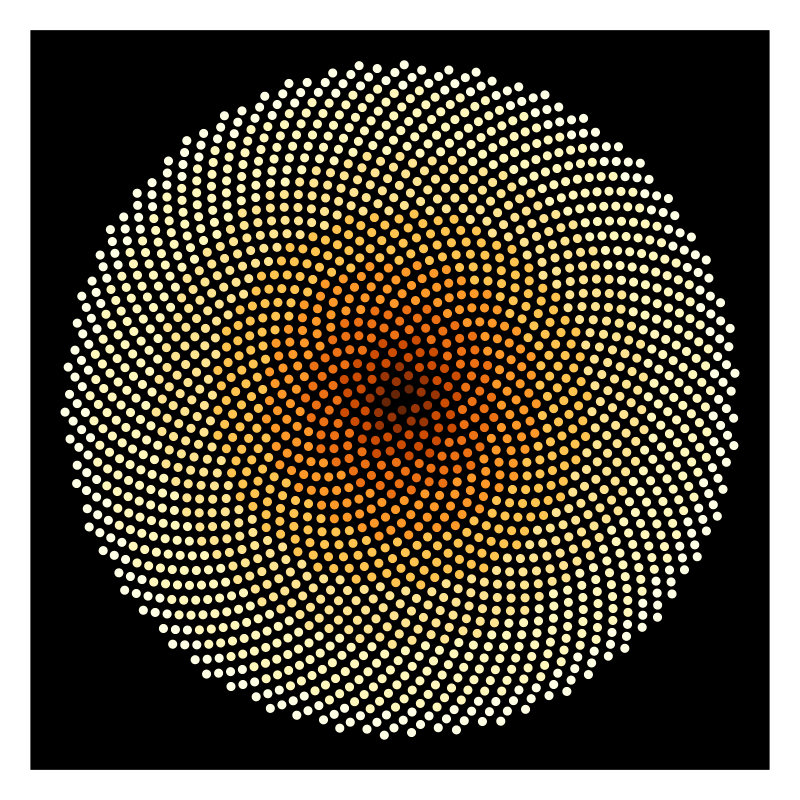H.Vogel’s pattern for sunflower forets.Author: Jeffrey Rosenbluth
> import Diagrams.Backend.SVG.CmdLine

A representation of Vogel’s model for the floret pattern of a sunflower head. Vogel, H (1979). “A better way to construct the sunflower head”. Mathematical Biosciences 44 (44): 179–189. doi:10.1016/0025-5564(79)90080-4.

> {-# LANGUAGE NoMonomorphismRestriction #-}
> import           Diagrams.Prelude
> import           Data.Colour.Palette.BrewerSet

The n florets of the sunflower are positioned at radii proportional to the square root of n and rotated by a factor 2.4 radians in accordance with Vogel’s model.

> mkCoords :: Int -> [P2 Double]
> mkCoords n =[coord (fromIntegral i) | i <- [1..n]]
>   where
>     coord m = p2 $fromPolar (sqrt m) (2.4 * m) > fromPolar r theta = (r * cos theta, r * sin theta) The color of each floret is based on it’s radius. > floret :: Double -> Diagram B > floret r = circle 0.6 # lw none # fc (colors !! n) > where > n = floor (1.4 * sqrt r) mod 10 > colors = black : (reverse$ brewerSet YlOrBr 9)
> sunflower :: Int ->  Diagram B
> sunflower n = frame 4 $position$ zip (mkCoords n) (florets n)
>   where
>     florets m = [floret (sqrt (fromIntegral i)) | i <- [1..m]]
> example :: Diagram B
> example = frame 4 \$ sunflower 2000 # bg black
> main = mainWith (example :: Diagram B)